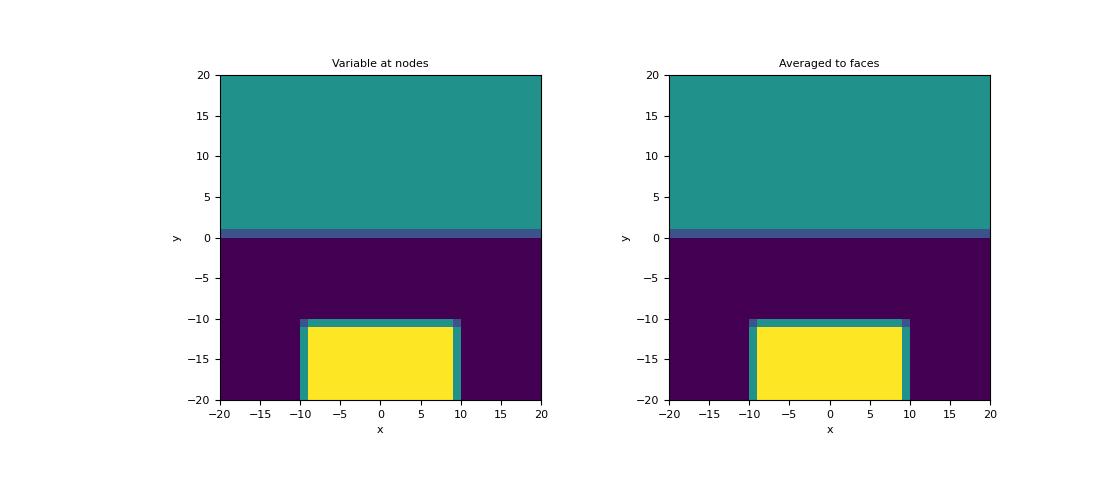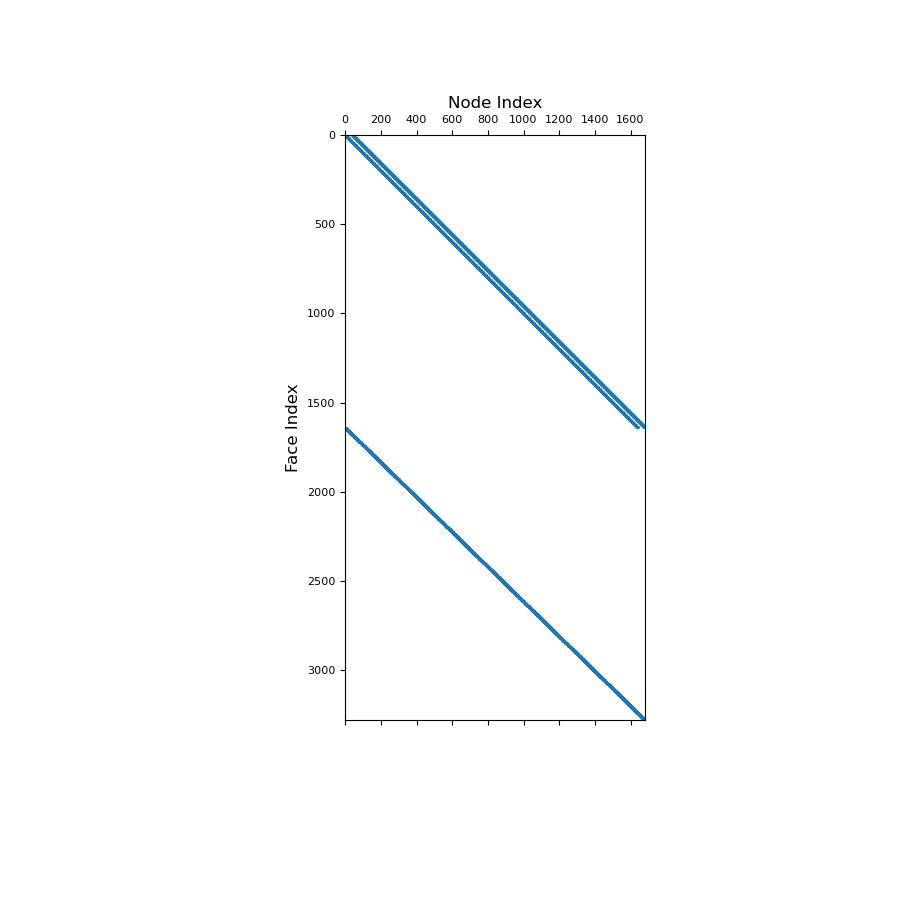# discretize.operators.DiffOperators.average_node_to_face¶

property DiffOperators.average_node_to_face

Averaging operator from nodes to faces (scalar quantities).

This property constructs a 2nd order averaging operator that maps scalar quantities from nodes to edges; scalar at faces is organized in a 1D numpy.array of the form [x-faces, y-faces, z-faces]. This averaging operator is used when a discrete scalar quantity defined on mesh nodes must be projected to faces. Once constructed, the operator is stored permanently as a property of the mesh. See notes.

Returns
(n_faces, n_nodes) scipy.sparse.csr_matrix

The scalar averaging operator from nodes to faces

Notes

Let $$\boldsymbol{\phi_n}$$ be a discrete scalar quantity that lives on mesh nodes. average_node_to_face constructs a discrete linear operator $$\mathbf{A_{nf}}$$ that projects $$\boldsymbol{\phi_n}$$ to faces, i.e.:

$\boldsymbol{\phi_f} = \mathbf{A_{nf}} \, \boldsymbol{\phi_n}$

where $$\boldsymbol{\phi_f}$$ approximates the value of the scalar quantity at faces. For each face, we are simply averaging the values at the nodes which outline the face. The operation is implemented as a matrix vector product, i.e.:

phi_f = Anf @ phi_n


Examples

Here we compute the values of a scalar function on the nodes. We then create an averaging operator to approximate the function at the faces. We choose to define a scalar function that is strongly discontinuous in some places to demonstrate how the averaging operator will smooth out discontinuities.

We start by importing the necessary packages and defining a mesh.

>>> from discretize import TensorMesh
>>> import numpy as np
>>> import matplotlib.pyplot as plt
>>> h = np.ones(40)
>>> mesh = TensorMesh([h, h], x0="CC")


Then we, create a scalar variable on nodes

>>> phi_n = np.zeros(mesh.nN)
>>> xy = mesh.nodes
>>> phi_n[(xy[:, 1] > 0)] = 25.0
>>> phi_n[(xy[:, 1] < -10.0) & (xy[:, 0] > -10.0) & (xy[:, 0] < 10.0)] = 50.0


Next, we construct the averaging operator and apply it to the discrete scalar quantity to approximate the value on the faces.

>>> Anf = mesh.average_node_to_face
>>> phi_f = Anf @ phi_n


Plot the results,

Expand to show scripting for plot
>>> fig = plt.figure(figsize=(11, 5))
>>> mesh.plot_image(phi_n, ax=ax1, v_type="N")
>>> ax1.set_title("Variable at nodes")
>>> mesh.plot_image(phi_f, ax=ax2, v_type="F")
>>> ax2.set_title("Averaged to faces")
>>> plt.show()Below, we show a spy plot illustrating the sparsity and mapping of the operator

Expand to show scripting for plot
>>> fig = plt.figure(figsize=(9, 9))